## 背景

2. 书签栏 javascript: 协议链接脚本：一键登录教务系统脚本生成器 2.0

## 思路

1. 获取图片信息
2. 将图片二值化
3. 把图片中不同的字符分割出来
4. 将分割的字符通过旋转的方式标准化
5. 验证码的训练与识别

## 图片信息的获取

let img = document.getElementById("icode");
// 获取图片宽高
const { width, height } = img;
// 通过 canvas 存图片
const canvas = document.createElement("canvas");
// 设置 canvas 的宽高
canvas.width = width;
canvas.height = height;
// context
const ctx = canvas.getContext("2d");

// 把验证码绘制到canvas中
ctx.drawImage(img, 0, 0);

// 提取像素颜色数组
let imageData = ctx.getImageData(0, 0, width, height).data;

## 二值化

// 下一步，二值化
let grayImageData = [];
for (let i = 0; i < 4 * width * height; i += 4) {
// 策略，蓝色优先
let white = imageData[i] < 105 && imageData[i + 1] < 105 && imageData[i + 2] > 100;
grayImageData.push(white ? 1 : 0);
}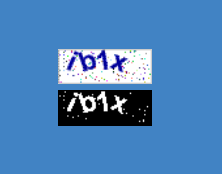// 获取坐标对应像素点的值
function get(x, y) {
return imageData[y * width + x];
}

// 设置像素点的值
function set(x, y, value) {
imageData[y * width + x] = value;
}

imageData.forEach((v, i) => {
// 第几行 i / width
// 第几列 i % width
let x = i % width, y = parseInt(i / width);
// console.log(x, ', ', y);
let u = get(x, y - 1) !== 0;
let d = get(x, y + 1) !== 0;
let l = get(x - 1, y) !== 0;
let r = get(x + 1, y) !== 0;
// 判断像素四周是否为孤立的像素点，没有就设置为0
// console.log(u + d + l + r);
if (v == 1 && (u + d + l + r) < 2) {
imageData[i] = 0;
}
});

// 定义一个 GrayImage 数据类型
function GrayImage(width, height, dataArr) {
this.width = width;
this.height = height;

// 预先设置图片数据
if (dataArr) {
this.imageData = dataArr;
} else {
this.imageData = [];
// 初始化：所有像素点置0
for (let i = 0; i < this.width * this.height; i++) {
this.imageData.push(0);
}
}
}

// 通过 Canvas API 把二值化图形可视化
GrayImage.prototype.draw = function (ctx) {
let imgArr = new Uint8ClampedArray(4 * this.width * this.height);
for (let i = 0; i < this.width * this.height; i++) {
for (let j = 0; j < 3; j++) {
imgArr[4 * i + j] = this.imageData[i] * 255;
}
imgArr[4 * i + 3] = 255;
}

let newImageData = new ImageData(imgArr, this.width, this.height);

ctx.putImageData(newImageData, 0, 0);
};

// 获取坐标对应像素点的值
GrayImage.prototype.get = function (x, y) {
return this.imageData[y * this.width + x];
};

// 设置像素点的值
GrayImage.prototype.set = function (x, y, value) {
this.imageData[y * this.width + x] = value;
};

// 去除孤立噪点
GrayImage.prototype.removeNoise = function () {
this.imageData.forEach((v, i) => {
// 第几行 i / width
// 第几列 i % width
let x = i % this.width, y = parseInt(i / this.width);
let u = this.get(x, y - 1) !== 0;
let d = this.get(x, y + 1) !== 0;
let l = this.get(x - 1, y) !== 0;
let r = this.get(x + 1, y) !== 0;
// 判断像素四周是否为孤立的像素点，没有就设置为0
if (v == 1 && (u + d + l + r) < 2) {
this.imageData[i] = 0;
}
});
};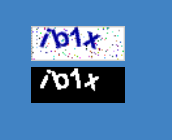## 分割字符串

// 去除四周空白部分
GrayImage.prototype.removeBlank = function () {
let newImageData = [];
// 上下左右边界
let u = this.height * 2;
let d = 0;
let l = -1;
let r = 0;
// 按竖线来扫描
for (let i = 0; i < this.width; i++) {
// 统计数量，方便区分左右边界
let count = 0;
for (let j = 0; j < this.height; j++) {
if (parseInt(this.get(i, j)) === 1) {
count++;
// 上边界
if (j < u) {
u = j;
}
// 下边界
if (j > d) {
d = j;
}
}
}
// 左边界，如果l没被赋值过就设置初值，以后都不变了
if (count > 0 && l === -1) {
l = i;
}
// 右边界
if (count > 0 && r < i) {
r = i;
}
}
// 若u没有改变，说明没扫到像素点
if (u === this.height * 2) {
u = 0;
}
// 保存到数组里面
for (let i = u; i < d + 1; i++) {
for (let j = l; j < r + 1; j++) {
// console.log(this.get(j, i));
newImageData.push(this.get(j, i));
}
}
this.imageData = newImageData;
// 新的宽高
this.width = r - l + 1;
this.height = d - u + 1;
}## 随机旋转字符的标准化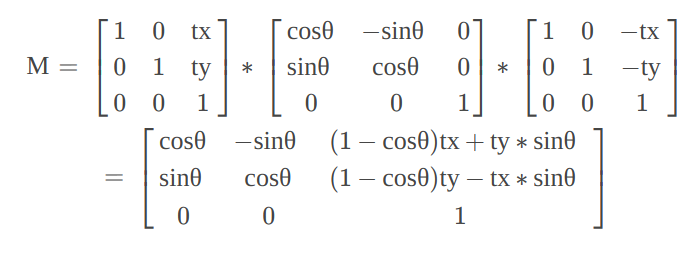function rotate(x, y, tx, ty, angle) {
let cos = Math.cos(angle);
let sin = Math.sin(angle);
let rx = Math.round(x * cos - y * sin + (1 - cos) * tx + sin * ty);
let ry = Math.round(x * sin + y * cos + (1 - cos) * ty - sin * tx);
return { rx, ry };
}

// 绕中心点旋转一定角度，返回一个旋转后的GrayImage
GrayImage.prototype.rotate = function (deg) {
// 创建一个 30 x 30 的临时画布
let grayChar = new GrayImage(30, 30);
// 开始旋转，像素矩阵需要经过旋转矩阵变换得到新的矩阵
let tx = Math.round(this.width / 2);
let ty = Math.round(this.height / 2);
for (let i = 0; i < this.width; i++) {
for (let j = 0; j < this.height; j++) {
let axis = rotate(i, j, tx, ty, deg * Math.PI / 180); // 旋转角，需要转换为弧度制
// console.log(i, j, axis);
// +8是为了把旋转后的图形平移到可视范围，方便进一步切割
grayChar.set(axis.rx + 8, axis.ry + 8, this.get(i, j));
}
}
// 返回之前去除多余空白
grayChar.removeBlank();
return grayChar;

//
// 旋转角度函数，基于齐次坐标的线性变换
// http://blog.csdn.net/csxiaoshui/article/details/65446125
//
function rotate(x, y, tx, ty, angle) {
let cos = Math.cos(angle);
let sin = Math.sin(angle);
let rx = Math.round(x * cos - y * sin + (1 - cos) * tx + sin * ty);
let ry = Math.round(x * sin + y * cos + (1 - cos) * ty - sin * tx);
return { rx, ry };
}
}

// 获得标准化字符（把歪了的字符转回来）
GrayImage.prototype.normalize = function () {
let charList = [];
let minIndex = -1;
let minWidth = this.width;
// 从-25度旋转到25度，比较宽度，找到宽度最小的一个
for (let i = -25; i <= 25; i += 2) {
let newChar = this.rotate(i);
charList.push(newChar);
if (newChar.width < minWidth) {
minWidth = newChar.width;
minIndex = charList.length - 1;
}
}
if (minIndex === -1)
return this;
// console.log(minWidth, minIndex);
return charList[minIndex];
};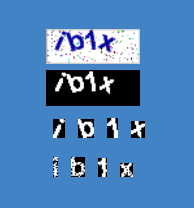## 样本训练与识别的实现

Levenshtein distance 算法本身在网上有许多实现，经过对比之后我选择了一个目前性能表现最好的一个库 gustf/js-levenshtein, 顺便用 Node 的 Express 框架实现了一个简单的人工打码样本收集器，将样本的宽高，对应字符，二值化数组数据保存在一个 SQLite 数据库中。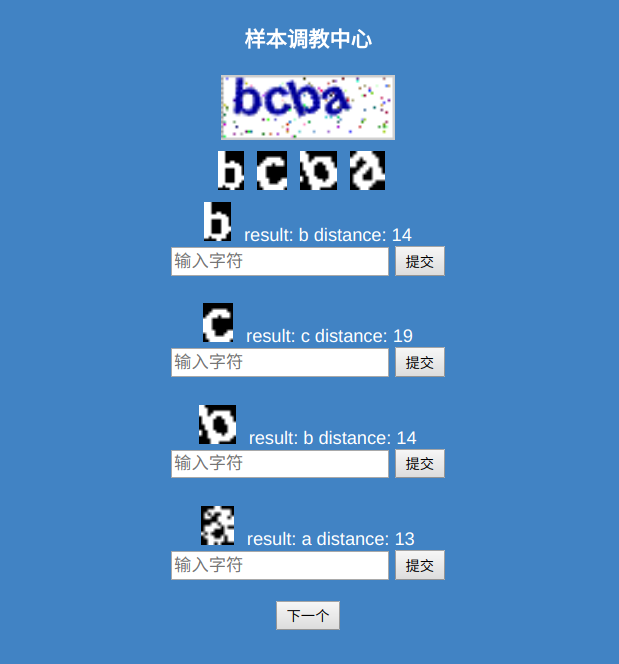// charList 样本库数据对象
let charList = {
// 按照宽度分类的字符列表
charQueueList: null,
// 从服务器下载样本集
try {
let { success, data, version } = await fetchURL("/chars/get");
if (!success) throw "error";
// 保存样本集的数组
let charList = data;
// 按照宽度来分类样本集
this.charQueueList = [];
charList.forEach((v) => {
// 创建二维数组
if (!this.charQueueList[v.width]) {
this.charQueueList[v.width] = [];
}
this.charQueueList[v.width].push(v);
});
// 存入缓存
storage.set("charListVersion", version);
storage.set("charList", this.charQueueList);
// 开始处理
return { version };
} catch (e) {
return Promise.reject(e);
}
},
// 找到与传入的 GrayImage 最接近的字符
recognize: function (char) {
console.log(char);
let distance;
let c;
// 取出宽度对应的执行队列
let queue = this.charQueueList[char.width];
// console.log(queue);
for (let i = 0; i < queue.length; i++) {
let d = levenshtein(char.imageData.join(""), queue[i].data);
if (distance === undefined || d < distance) {
distance = d;
c = queue[i];
}
if (distance < 4) break;
}

return { distance, char: c };
},
}

## 后记

1.Lesun

刚好我们学校也是正方的系统，但是我已经用不上选课了，推荐给学弟学妹

1.qing

我也快用不上了哈哈哈哈

2.坤的

不错!虽然用不上.